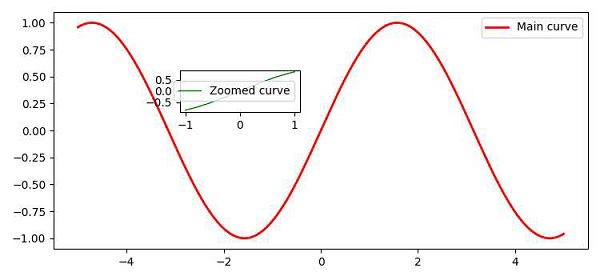# How to zoom a portion of an image and insert in the same plot in Matplotlib?

To zoom a portion of an image and insert in the same plot, we can take the following steps −

• Create x and y points, using numpy.

• To zoom a part of an image, we can make data for x and y points, in that range.

• Plot x and y points (Step 1), using the plot() method with lw=2, color red and label.

• Use the legend() method to place text for the plot, Main curve.

• Create the axes using the axes() method by putting the rectangle’s coordinate.

• Plot x and y points (Step 2), using the plot() method with lw=1, color='green' and label, i.e., subpart of the plot.

• Use the legend() method to place text for the plot, zoomed curve.

• To display the figure, use the show() method.

## Example

import numpy as np
from matplotlib import pyplot as plt
plt.rcParams["figure.figsize"] = [7.50, 3.50]
plt.rcParams["figure.autolayout"] = True
x = np.linspace(-5, 5, 1000)
y = np.sin(x)
x_zoom = np.linspace(-1, 1, 50)
y_zoom = np.sin(x_zoom)
plt.plot(x, y, c='red', lw=2, label="Main curve")
plt.legend()
axes = plt.axes([.30, .6, .20, .15])
axes.plot(x_zoom, y_zoom, c='green', lw=1, label="Zoomed curve")
axes.legend()
plt.show()

## Output